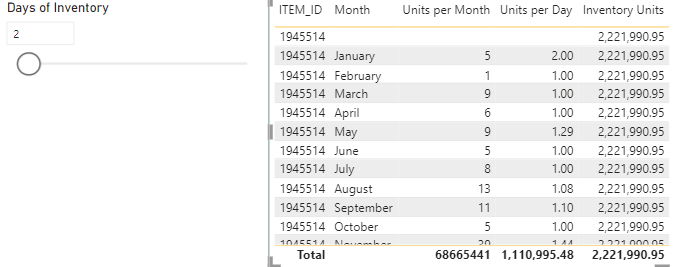cancel
Showing results for
Did you mean:Helper III

## Selected Value Times Each Row?

Hi all

I am trying to create some kind of formula that would work based on a selected value/what if parameter. My what if parameter is how many days of stock I want to have on hand - it is whole numbers from 1 - 60.

Then I have created a summary table that has Item ID, Month, Average items sold per day, and total items sold during that month. I want to create a formula that multiplies the average number of items sold per day by the stock days on hand (what if parameter). Here is what I've tried -

Inventory Units =
VAR InternalTable = summarize('Order Data','Order Data'[ITEM_ID],'Order Data'[Month], "Units per Day", sum('Order Data'[ORIG_ORDER_QTY])/DISTINCTCOUNT('Order Data'[Date Time]), "Units per Month", sum('Order Data'[ORIG_ORDER_QTY]))

VAR InvUnits = sumx(InternalTable, [Units per Day] * SELECTEDVALUE('Days of Inventory'[Days of Inventory]))

RETURN
InvUnits

This is just returning blanks for each row. Any help would be greatly appreciated!
1 ACCEPTED SOLUTIONSuper User

Try

``````Inventory Units =
DIVIDE (
SUM ( 'Order Data'[ORIG_ORDER_QTY] ),
DISTINCTCOUNT ( 'Order Data'[Date Time] )
)
* SELECTEDVALUE ( 'Days of Inventory'[Days of Inventory] )``````
7 REPLIES 7Super User

What is the relationship between the two tables? How does your visual look like?Helper III

Between which two tables? The summary table? Right now I only have the one table and the what-if parameter, which I have not connected to anywhere. The Days of Inventory is a what-if parameter that simply contains numbers between 1-60.Super User

That is strage. Must be something in the visual. But ofcaurse you select only value right?  Can you please share a screenshotHelper III

I officially got it to not be blank, but now it's all the same value -Super User

Try

``````Inventory Units =
DIVIDE (
SUM ( 'Order Data'[ORIG_ORDER_QTY] ),
DISTINCTCOUNT ( 'Order Data'[Date Time] )
)
* SELECTEDVALUE ( 'Days of Inventory'[Days of Inventory] )``````Helper III

I think this worked! Double checking it now manually to make sure, but it looks good!Community Support

Hi @Thigs ,

May I ask if your problem has been solved? Did tamerj1's post above help you? If any of the posts are helpful, then please consider accepting it as a solution to help other members find it faster. Many thanks!

Best Regards,
Community Support Team_Gao